# Pie Charts with D3.js

Pie charts are one of many visualizations that you can create using D3.js. We are going to learn how to do the following in this post.

• Make a circle
• Make a donut
• Make a pie wedge
• Make a segment
• Make a pie chart

Most of these examples require just a minor adjustment in a standard piece of code. This  will make more sense in a minute.Make a Circle

Making a circle involves using the .arc() method. In this method there are four parameters that you manipulate to get different shapes. They are explained below

• .innerRadius() This parameter makes a whole in your circle to give the appearance of a donut
• .startAngle() is used in combination with .endAngle() to make a pie wedge

Therefore, to make several different shapes we manipulate these different parameters. In the code below, we create the svg element first (lines 7-10)  then our circle (lines 11-15). Lastly, we append the path to the svg element (lines 16-21). Below is the code and picture.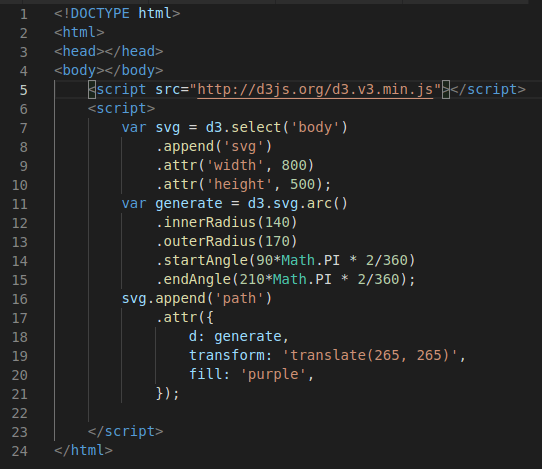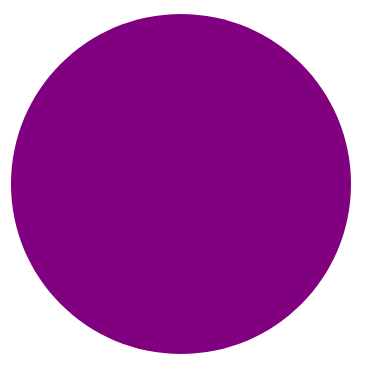The rest of the examples primarily deal with manipulating the existing code.

Donut

To make the donut, you need to change the value inside the .innerRadius() parameter. The larger the value the bigger the hole in the middle of the circle will become. In order to generate the donut below you need to change the value found in line 12 of  the code to 100.Pie Wedge

To make a wedge you need to replace lines 14-15 of the code with the following

```.startAngle(0*Math.PI * 2/360)

.endAngle(90*Math.PI * 2/360);```

This is telling d3.js to start the angle at 0 degrees and stop at 90 degrees. This is another way of saying making a wedge of 1/4 of the circle. Doing this will create the following.Segment

In order to make the segment you keep the code the same as above for the pie wedge but at a change to the .innerRadius() parameter. AS shown below,

```.innerRadius(100)

.startAngle(0*Math.PI * 2/360)

.endAngle(90*Math.PI * 2/360);```Pie Chart

A pie chart is just a more complex version of what we have already done. You still need to set up your svg element. This is don in lines 7-14. Notice that we also had to add a g element and a transform attribute.

Line 16 contains the data for making the pie chat. This is hard coded but we can also use other forms of data. Line 17 uses the .pie() method with the data to set the stage for our pie chart.

Lines 19-27 are for generating the arc. This code is mostly the same except for the functions that are used for the .startAngle() and .end Angle() methods. Line 29 sets the color and Lines 30-42 draw the paths for creating the image. The code with the // will be explained in a moment. Below is the code and the pie chart.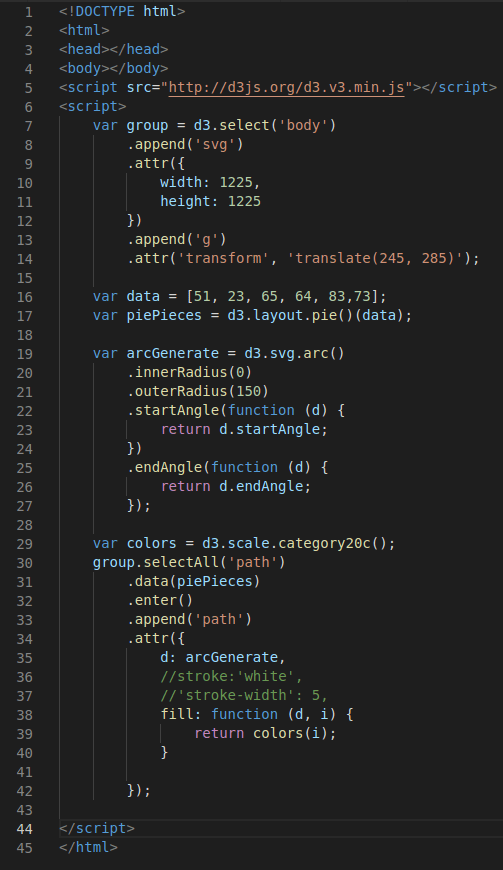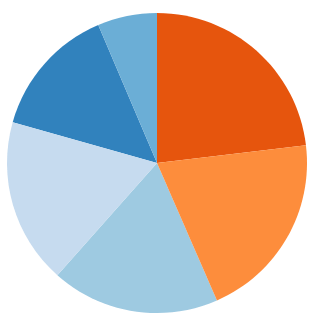Pie Chart Variation

Below is the same pie chart but with some different features.

• It now has a donut (line 20 change .innerRadius(0) to .innerRadius(50))
• There are now separations between the different segments to give the appearance that it is pulling apart. Remove the // in lines 36-37 to activate this.

Below is the pie chart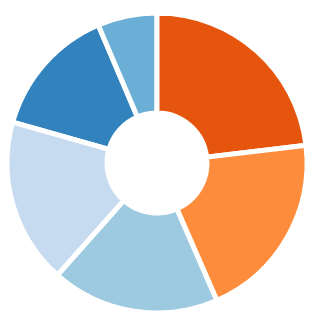You can perhaps now see that the possibilities are endless with this.

Conclusion

Pie charts and their related visualizations are another option for communicating insights of data